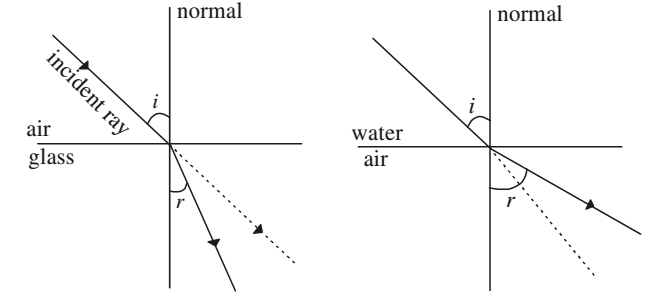# Refraction of Light

When light passes obliquely from a rarer medium (air) to a denser medium (water, glass), there is a change in its direction of propagation. This bending of light at the boundary of two dissimilar media is called refraction.

When a ray of light is refracted at an interface, it obeys the following two laws:

Law I: The incident ray, the refracted ray and the normal to the surface at the point of incidence always lie in the same plane.

Law II: The ratio of the sine of the angle of incidence to the sine of the angle of refraction is constant for a given pair of media. It is independent of the angle of incidence when light propagates from a rarer to a denser medium. Moreover, for a light of given color, the ratio depends only on the pair of media.

This law is referred to as Snell’s law. According to Snell’s law

(sin i)/(sin r) = µ12

where µ12 is a constant, called the refractive index of second medium with respect to the first medium, and determines how much bending would take place at the interface separating the two media. It is also expressed as the ratio of the velocity of light in the first medium to the velocity of light in the second medium.

The refracted ray bends towards the normal on the air-glass interface and bends away from the normal on water-air interface.